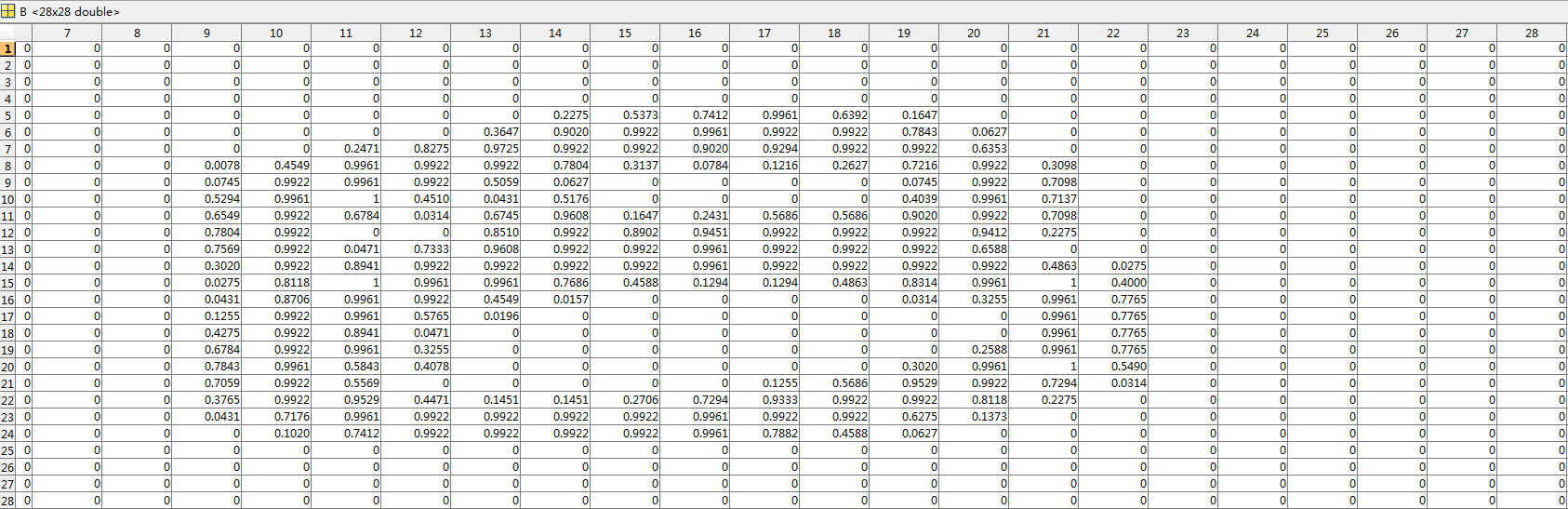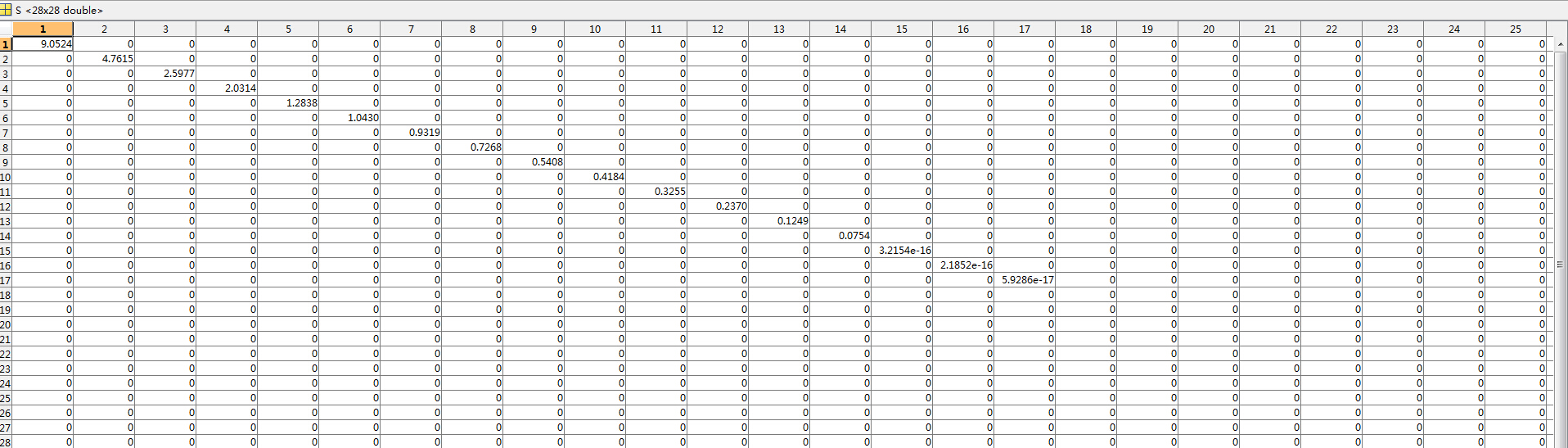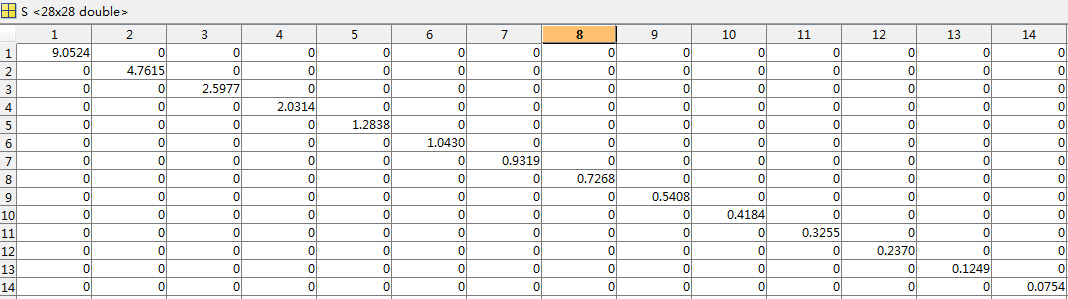# 简单易学的机器学习算法——SVD奇异值分解

## 一、SVD奇异值分解的定义

假设是一个的矩阵，如果存在一个分解：

# 二、SVD奇异值分解与特征值分解的关系

特征值分解与SVD奇异值分解的目的都是提取一个矩阵最重要的特征。然而，特征值分解只适用于方阵，而SVD奇异值分解适用于任意的矩阵，不一定是方阵。

# 三、SVD奇异值分解的作用和意义

奇异值分解最大的作用就是数据的降维，当然，还有其他很多的作用，这里主要讨论数据的降维，对于的矩阵，进行奇异值分解

# 五、实验的仿真

我们在手写体上做实验，原始矩阵为MATLAB代码
%% 测试奇异值分解过程
B = zeros(28,28);%将行向量重新转换成原始的图片

for i = 1:28
j = 28*(i-1)+1;
B(i,:) = A(1,j:j+27);
end

%进行奇异值分解
[U S V] = svd(B);

%选取前面14个非零奇异值
for i = 1:14
for j = 1:14
S_1(i,j) = S(i,j);
end
end

%左奇异矩阵
for i = 1:28
for j = 1:14
U_1(i,j) = U(i,j);
end
end

%右奇异矩阵
for i = 1:28
for j = 1:14
V_1(i,j) = V(i,j);
end
end

B_1 = U_1*S_1*V_1';

%同时输出两个图片
subplot(121);imshow(B);
subplot(122);imshow(B_1);


©️2019 CSDN 皮肤主题: 编程工作室 设计师: CSDN官方博客